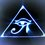# Trigonometric Identities In Right Angle TrianglesIn right angle triangles there is one 90 degree angle and two other angles that are said to be complementary angles on the basis that the sum of interior angles of a triangle is 180 degrees.

Since the two angles A and B are complementary angles they have special properties that allow us to manipulate certain trigonometric identities for right angle triangles. I originally noticed this while I was looking at compound angle formulas and realized that if the angles are complementary then it results in the co-function identities. I then appplied this theory to the Pythagorean identities and proved a new identity for right angle triangles.

The pythagorean identity states that:

sin^2(A)+cos^2(A)=1

sin^2(B)+cos^2(B)=1

In a right angle triangle this translates to:

sin^2(A)+sin^2(B)=1

cos^2(A)+cos^2(B)=1Note by Brody Acquilano
6 years, 7 months ago

This discussion board is a place to discuss our Daily Challenges and the math and science related to those challenges. Explanations are more than just a solution — they should explain the steps and thinking strategies that you used to obtain the solution. Comments should further the discussion of math and science.

When posting on Brilliant:

• Use the emojis to react to an explanation, whether you're congratulating a job well done , or just really confused .
• Ask specific questions about the challenge or the steps in somebody's explanation. Well-posed questions can add a lot to the discussion, but posting "I don't understand!" doesn't help anyone.
• Try to contribute something new to the discussion, whether it is an extension, generalization or other idea related to the challenge.

MarkdownAppears as
*italics* or _italics_ italics
**bold** or __bold__ bold
- bulleted- list
• bulleted
• list
1. numbered2. list
1. numbered
2. list
Note: you must add a full line of space before and after lists for them to show up correctly
paragraph 1paragraph 2

paragraph 1

paragraph 2

[example link](https://brilliant.org)example link
> This is a quote
This is a quote
    # I indented these lines
# 4 spaces, and now they show
# up as a code block.

print "hello world"
# I indented these lines
# 4 spaces, and now they show
# up as a code block.

print "hello world"
MathAppears as
Remember to wrap math in $$ ... $$ or $ ... $ to ensure proper formatting.
2 \times 3 $2 \times 3$
2^{34} $2^{34}$
a_{i-1} $a_{i-1}$
\frac{2}{3} $\frac{2}{3}$
\sqrt{2} $\sqrt{2}$
\sum_{i=1}^3 $\sum_{i=1}^3$
\sin \theta $\sin \theta$
\boxed{123} $\boxed{123}$

Sort by:

Are you sure that $\sin ^2 A + \cos^2 B = 1$? Why?
Shouldn't it be $\sin^2 \theta + \cos^2 \theta = 1$?

Staff - 6 years, 7 months ago

oops yes i will have to edit that, thanks!

- 6 years, 7 months ago